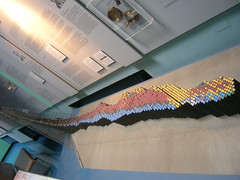Tag Archives: Hubble’s law
Aside

## Astrophysics and Cosmology

2 SepIntended as a replacement for File:Universe.svg and File:Universos.gif. Improvements: better dash patterns, more accurate curves (actual solutions of the Friedmann equations, not hand-drawn). (Photo credit: Wikipedia)Curve of Binding Energy Gamowtal Deutsches Museum (Photo credit: brewbooks)The path of a star with the mass of the Sun over Hertzsprung-Russell diagram during stellar evolution. (Photo credit: Wikipedia)The Big Crunch. The vertical axis can be considered as either plus or minus time. (Photo credit: Wikipedia)English: Graph of a function of total emitted energy of a black body j^{\star} proportional to the fourth power of its thermodynamic temeprature T , according to the Stefan–Boltzmann law. In blue is a total energy according to the Wien approximation, equals j^{\star} / \zeta(4) \approx 0.923938 j^{\star} . (Photo credit: Wikipedia)

1. use the expression F = Gm1m2/r2
2. derive and use the expression g = −Gm/r2 for the gravitational field due to a point mass
3. recall similarities and differences between electric and gravitational fields
4. recognize and use the expression relating flux, luminosity and distance F = L/4πd2 and the application to standard candles
5. explain how distances can be determined using trigonometric parallax and by measurements on radiation flux received from objects of known luminosity (standard candles)
6. Recognize and use a simple Hertzsprung-Russell diagram to relate luminosity and temperature. Use this diagram to explain the life cycle of stars
7. Recognize and use the expression L = σT4 x surface area, (for a sphere L = 4πr2σT4) (Stefan-Boltzmann law) for black body radiators.
8. recognize and use the expression: λmaxT = 2.898 x 10-3 m K (Wien’s law) for black body radiators
9. recognize and use the expressions z = Δλ/λ ≈ Δf/f ≈ v/c for a source of electromagnetic radiation moving relative to an observer and v = H0d for objects at cosmological distances
10. be aware of the controversy over the age and ultimate fate of the universe associated with the value of the Hubble Constant and the possible existence of dark matter
11. explain the concept of nuclear binding energy, and recognise and use the expression ΔE = c2Δm and use the non SI atomic mass unit (u) in calculations of nuclear mass (including mass deficit) and energy
12. describe the processes of nuclear fusion and fission
13. explain the mechanism of nuclear fusion and the need for high densities of matter and high temperatures to bring it about and maintain it
14.